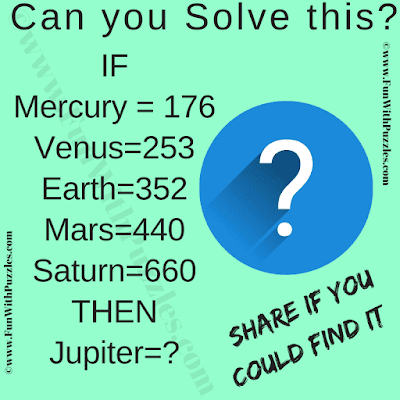This crack the code puzzle is only for genius minds. In this code-cracking puzzle, you are shown some equations. In these equations, the left side of the equation is the name of the Planet in our solar system. However, the right side of the equation contains a number. Your challenge in this genius puzzle is to crack the code which relates the planet names to the numbers. Once you are able to crack this code, then find the value of the missing number which will replace the question mark in the last equation.Can you Crack the Code?

The answer to this "Crack the Code Puzzle", can be viewed by clicking on the answer button.